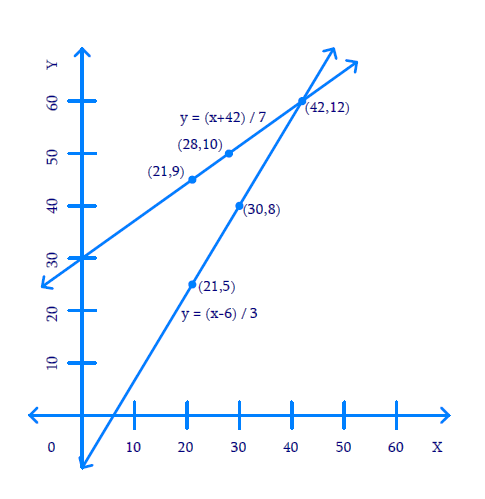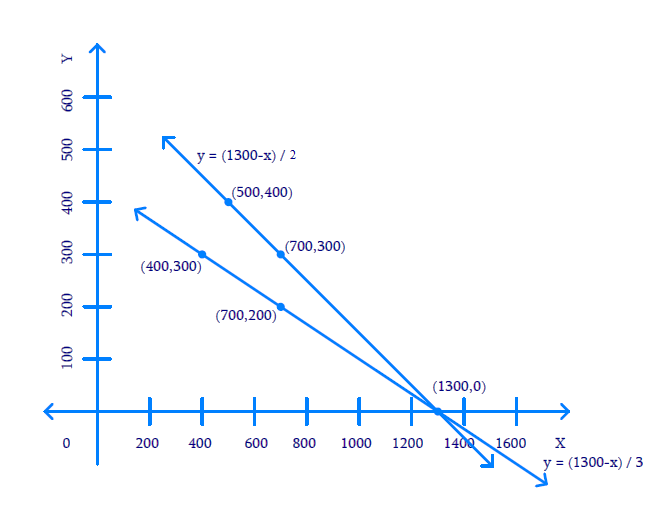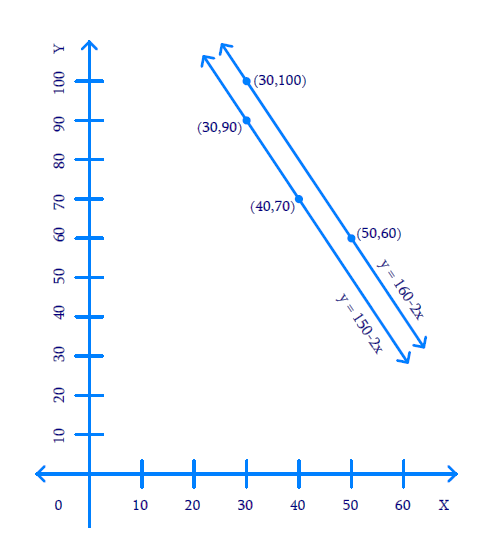NCERT Class 10 Maths Pair of Linear Equations in Two Variables

# NCERT Class 10 Maths Pair of Linear Equations in Two Variables

The introduction part of the chapter 3 presents a fact that each solution (x, y) of a linear equation in two variables, ax + by + c = 0, corresponds to a point on the line representing the equation, and vice versa. Next, a pair of linear equations in two variables is explained as a combination of two linear equations that are in the same two variables x and y. Next, several solved examples are presented wherein students are told about the graphical representation of linear equations in two variables. Thereafter, for the second exercise, the graphical method of solving a pair of linear equations in two variables is presented. The rest of the chapter covers concepts like - algebraic method of solving a pair of linear equations in two variables, elimination method, cross-multiplication method, and equations reducible to a pair of linear equations in two variables.

Download FREE PDF of Chapter-3 Pair of Linear Equations in Two Variables

## Chapter 3 Ex.3.1 Question 1

Aftab tells his daughter, “Seven years ago, I was seven times as old as you were then. Also, three years from now, I shall be three times as old as you will be.” (Isn’t this interesting?) Represent this situation algebraically and graphically.

### Solution

What is Known?

$$7$$ years ago, Aftab was $$7$$ times as old as his daughter then and $$3$$ years from now, Aftab shall be $$3$$ times as old as his daughter will be.

What is Unknown?

Represent the situation algebraically and graphically.

Reasoning:

Assume the present age of Aftab be $$x$$  years and his daughter be $$y$$ years then represent their ages $$7$$ years later and $$3$$ years ago in term of $$x$$ and $$y.$$ Two linear equations can be formed to represent the above situation algebraically.

Using algebraic equation and truth table they can be graphically represented.

Steps:

(i) Present age of Aftab $$= x$$ years and his daughter $$= y$$ years

Therefore, $$7$$ years ago, age of Aftab $$= \left( {x - 7} \right)$$ years and his daughter $$= \left( {y - 7} \right)$$years

Using this information and applying the known condition that $$7$$ years ago, Aftab was $$7$$ times as old as his daughter then:

\begin{align}x - 7 &= 7(y - 7)\\x - 7 &= 7y - 49\\x - 7y - 7 + 49 &= 0\\x - 7y + 42 &= 0\end{align}

After $$3$$ years from now, age of Aftab $$= \left( {x + 3} \right)$$ years and his daughter $$= \left( {y + 3} \right)$$ years and also Aftab will be $$3$$ times as old as his daughter. Then mathematically,

\begin{align}x + 3 &= 3\left( {y + 3} \right)\\x + 3 &= 3y + 9\\x - 3y + 3 - 9 &= 0\\x - 3y - 6 &= 0\end{align}

Algebraic representations, where $$x$$ and $$y$$ are present ages of Aftab and his daughter respectively:

\begin{align}x - 7y + 42 &= 0\qquad(1)\\x - 3y-6 &= 0\qquad(2)\end{align}

Therefore, the algebraic representation is for equation $$1$$ is:

\begin{align}x - 7y + 42 &= 0\\- 7y &= - x - 42\\7y &= x + 42\\y &= \frac{{x + 42}}{7}\end{align}

And, algebraic representation for equation $$(2)$$ is:

\begin{align}x - 3y - 6 &= 0\\- 3y &= - x + 6\\3y &= x - 6\\y &= \frac{{x - 6}}{3}\end{align}

Let us represent these equations graphically. For this, we need at least two solutions for each equation. We give these solutions in table shown below.

For equation $$(1)$$

 $$x$$ $$21$$ $$28$$ $$y = \frac{{x + 42}}{7}$$ $$9$$ $$10$$

For equation (2)

 $$x$$ $$30$$ $$21$$ $$y = \frac{{x - 6}}{3}$$ $$8$$ $$5$$

The graphical representation is as follows.Unit: $$1 \,\rm{cm}=5$$ years.

The answer is $$(42, \;12)$$

## Chapter 3 Ex.3.1 Question 2

The coach of a cricket team buys $$3$$ bats and 6 balls for $$₹\,3900.$$ Later, she buys another bat and $$3$$ more balls of the same kind for ₹ $$1300.$$ Represent this situation algebraically and geometrically.

### Solution

What is Known?

(i) Three bats and six balls for ₹ $$3900$$

(ii) One bat and three balls for ₹ $$1300$$

What is Unknown?

Represent the situation geometrically and algebraically

Reasoning:

Assuming the cost of one bat as ₹ $$x$$ and the cost of one ball as ₹ $$y,$$ two linear equations can be formed for the above situation.

Steps:

The cost of $$3$$ bats and 6 balls is ₹ $$3900.$$

Mathematically:

\begin{align}3x + 6y&= 3900\\3(x + 2y)& = 3900\\x + 2y& = 1300\end{align}

Also, the cost of $$1$$ bat and $$3$$ balls is ₹ $$1300.$$

Mathematically:

$x + 3y = 1300$

Algebraic representation where $$x$$ and $$y$$ are cost of bat and ball respectively.

\begin{align}x + 2y &= 1300 \qquad(1)\\x + 3y &= 1300\qquad(2)\end{align}

Therefore, the algebraic representation for equation $$1$$ is:

\begin{align}x + 2y &= 1300\\2y &= 1300-x\\y &= \frac{{1300 - x}}{2}\end{align}

And, the algebraic representation for equation $$2$$ is:

\begin{align}x + 3y &= 1300\\3y &= 1300-x\\y &= \frac{{1300 - x}}{3}\end{align}

Let us represent these equations graphically. For this, we need at least two solutions for each equation. We give these solutions in table shown below.

 $$x$$ $$700$$ $$500$$ $$y = \frac{{1300 - x}}{2}$$ $$300$$ $$400$$

 $$x$$ $$400$$ $$700$$ $$y = \frac{{1300 - x}}{3}$$ $$300$$ $$200$$

The graphical representation is as follows.Unit: $$1\,\rm{cm} =$$$$100.$$

The answer is $$(1300, \,0)$$

## Chapter 3 Ex.3.1 Question 3

The cost of $$2 \,\rm{kg}$$ of apples and $$1 \,\rm{kg}$$ of grapes on a day was found to be ₹ $$160$$ After a month, the cost of $$4\,\rm{kg}$$ of apples and $$2\,\rm{kg}$$ of grapes is ₹ $$300$$ Represent the situation algebraically and geometrically.

### Solution

What is Known?

(i) Cost of $$2 \,\rm{kg}$$of apples and $$1 \,\rm{kg}$$ of grapes is ₹ $$160$$

(ii) Cost of $$4 \,\rm{kg}$$ of apples and $$2 \,\rm{kg}$$ of grapes is ₹ $$300.$$

What is Unknown?

Represent the situation geometrically and algebraically.

Reasoning:

Assuming the cost of $$1 \,\rm{kg}$$ apples as ₹ $$x$$ and the cost of $$1 \,\rm{kg}$$ grapes as ₹ $$y,$$ two linear equations can be formed for the above situation.

Steps:

Let the cost of $$1 \,\rm{kg}$$ of apples be $$x$$ and cost of $$1 \,\rm{kg}$$ of grapes be $$y$$

Cost kg $$2 \,\rm{kg}$$ apples and $$1 \,\rm{kg}$$ of grapes is ₹ $$160.$$

Mathematically,

$2x + y = 160$

Also, cost kg $$4 \,\rm{kg}$$ apples and $$2 \,\rm{kg}$$ of grapes is ₹ $$300.$$

Mathematically,

\begin{align}4x + 2y &= 300\\2(2x + y) &= 300\\2x\, + \,y\,& = \,150\end{align}

Algebraic representation where $$x$$ and $$y$$ are the cost of $$1 \,\rm{kg}$$ apple and $$1 \,\rm{kg}$$ grapes respectively.

\begin{align}2x + y &= 160 \,\dots(1)\\2x + y& = 150\,\dots(2)\end{align}

Therefore, the algebraic representation is for equation $$1$$ is:

\begin{align}2x + y &= 160\\y &= 160-2x\end{align}

And, the algebraic representation is for equation $$2$$ is:

\begin{align}2x + y& = 150\\y &= 150-2x\end{align}

Let us represent these equations graphically. For this, we need at least two solutions for each equation. We give these solutions in table shown below.

 $$x$$ $$50$$ $$30$$ $$y = 160-2x$$ $$60$$ $$100$$

 $$x$$ $$30$$ $$40$$ $$y = 150-2x$$ $$90$$ $$70$$

The graphical representation is as follows.Unit $$= 1\, \rm{cm} =$$$$10$$

Since the lines are parallel hence no Solution

Pair of Linear Equations in Two Variables | NCERT Solutions
Instant doubt clearing with Cuemath Advanced Math Program